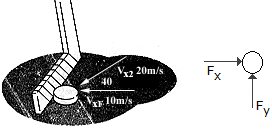# Engineering Mechanics - KOP: Impulse and Momentum - Discussion

### Discussion :: KOP: Impulse and Momentum - General Questions (Q.No.17)

17.A hockey puck is traveling to the left with a velocity of v1 = 10 m/s when it is struck by a hockey stick and given a velocity of v2 = 20 m/s as shown. Determine the magnitude of the net impulse exerted by the hockey stick on the puck. The puck has a mass of 0.2 kg.

 [A]. Imp = 6.00 N-s [B]. Imp = 2.00 N-s [C]. Imp = 2.78 N-s [D]. Imp = 5.68 N-s

Explanation:

No answer description available for this question.

 Bapmon said: (Oct 25, 2016) To answer this question you take the velocity in the x, and the velocity in the y. Take the magnitude and multiply by the mass right!